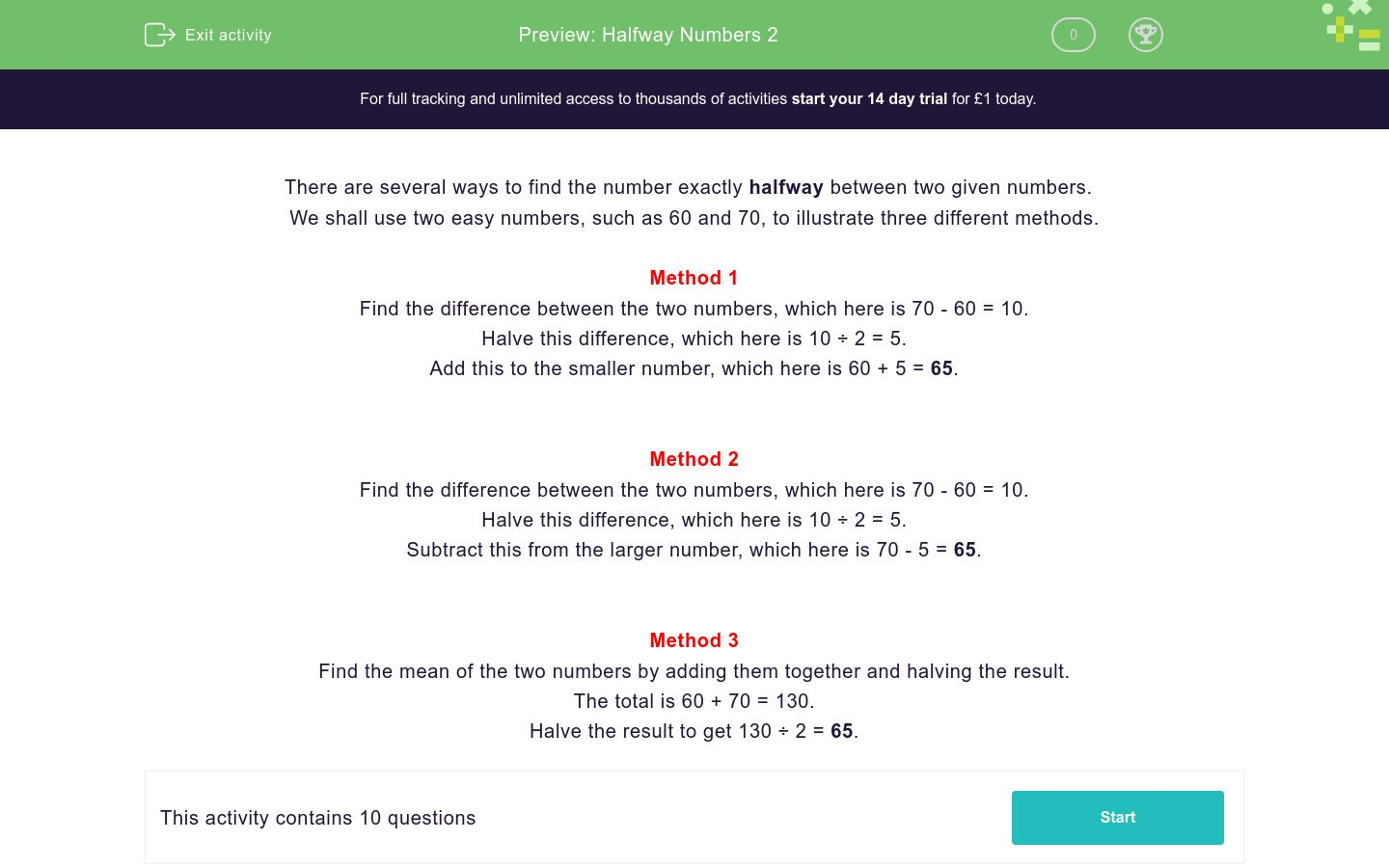# Halfway Numbers 2

In this worksheet, students must find the number which is exactly halfway between the two given numbers.Key stage:  KS 2

Curriculum topic:   Number: Addition, Subtraction, Multiplication and Division

Curriculum subtopic:   Perform Mental Calculations

Difficulty level:### QUESTION 1 of 10

There are several ways to find the number exactly halfway between two given numbers.

We shall use two easy numbers, such as 60 and 70, to illustrate three different methods.

Method 1

Find the difference between the two numbers, which here is 70 - 60 = 10.

Halve this difference, which here is 10 ÷ 2 = 5.

Add this to the smaller number, which here is 60 + 5 = 65.

Method 2

Find the difference between the two numbers, which here is 70 - 60 = 10.

Halve this difference, which here is 10 ÷ 2 = 5.

Subtract this from the larger number, which here is 70 - 5 = 65.

Method 3

Find the mean of the two numbers by adding them together and halving the result.

The total is 60 + 70 = 130.

Halve the result to get 130 ÷ 2 = 65.

Write down the number which is halfway between:

15400 and 19400

Write down the number which is halfway between:

5000 and 9480

Write down the number which is halfway between:

5420 and 5500

Write down the number which is halfway between:

5000 and 7420

Write down the number which is halfway between:

52120 and 63000

Write down the number which is halfway between:

54132 and 64132

Write down the number which is halfway between:

25400 and 10400

Write down the number which is halfway between:

15400 and 18460

Write down the number which is halfway between:

54140 and 74680

Write down the number which is halfway between:

540100 and 640300

• Question 1

Write down the number which is halfway between:

15400 and 19400

17400
EDDIE SAYS
Difference is 4000.
Halve this to get 2000.
• Question 2

Write down the number which is halfway between:

5000 and 9480

7240
EDDIE SAYS
Difference is 4480.
Halve this to get 2240.
• Question 3

Write down the number which is halfway between:

5420 and 5500

5460
EDDIE SAYS
Difference is 80.
Halve this to get 40.
• Question 4

Write down the number which is halfway between:

5000 and 7420

6210
EDDIE SAYS
Difference is 2420.
Halve this to get 1210.
• Question 5

Write down the number which is halfway between:

52120 and 63000

57560
EDDIE SAYS
Difference is 63000 - 52120 = 10880.
Halve this to get 5440.
• Question 6

Write down the number which is halfway between:

54132 and 64132

59132
EDDIE SAYS
Difference is 10000.
Halve this to get 5000.
• Question 7

Write down the number which is halfway between:

25400 and 10400

17900
EDDIE SAYS
Difference is 25400 - 10400 = 15000.
Halve this to get 7500.
• Question 8

Write down the number which is halfway between:

15400 and 18460

16930
EDDIE SAYS
Difference is 18460 - 15400 = 3060.
Halve this to get 1530.
• Question 9

Write down the number which is halfway between:

54140 and 74680

64410
EDDIE SAYS
74680 + 54140 = 128820.
Half this to get 64410.
• Question 10

Write down the number which is halfway between:

540100 and 640300

590200
EDDIE SAYS
Difference is 640300 - 540100 = 100200.
Halve this to get 50100.